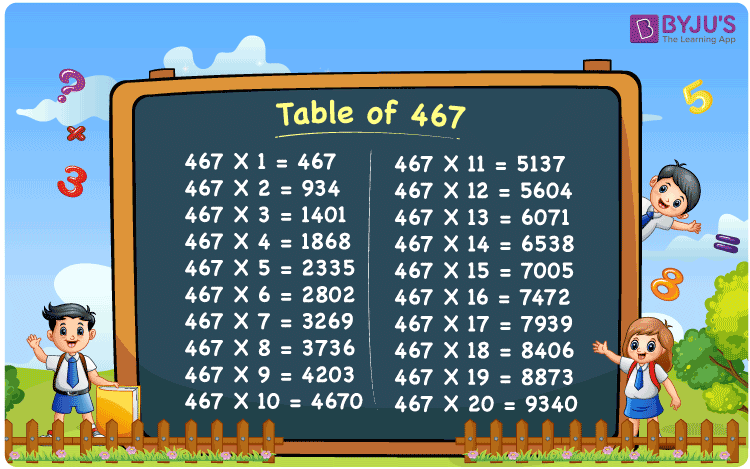Checkout JEE MAINS 2022 Question Paper Analysis : Checkout JEE MAINS 2022 Question Paper Analysis :

# Table of 467

The table of 467 is the multiplication table representing the multiples of the original number. These multiples can be produced by multiplying 467 to the set of natural numbers. The 467 times table indicates the repeated addition of 467 to itself when it is multiplied by any number. Students can use the table of 467 to solve problems related to the multiplication of 467. Learn more about multiplication tables here at BYJU’S. Students can also download the PDF of table 467 to retain it offline.

## Table of 467 Chart## What is the 467 Times Table?

As we already mentioned, the table of 467 represents the repeated addition of the number 467 when multiplied by any other number. Let us learn how we can make the 467 times table.

 Using multiplication Using repeated addition 467 × 1 = 467 467 467 × 2 = 934 467 + 467 = 934 467 × 3 = 1401 467 + 467 + 467 = 1401 467 × 4 = 1868 467 + 467 + 467 + 467 = 1868 467 × 5 = 2335 467 + 467 + 467 + 467 + 467 = 2335 467 × 6 = 2802 467 + 467 + 467 + 467 + 467 + 467 = 2802 467 × 7 = 3269 467 + 467 + 467 + 467 + 467 + 467 + 467 = 3269 467 × 8 = 3736 467 + 467 + 467 + 467 + 467 + 467 + 467 + 467 = 3736 467 × 9 = 4203 467 + 467 + 467 + 467 + 467 + 467 + 467 + 467 + 467 = 4203 467 × 10 = 4670 467 + 467 + 467 + 467 + 467 + 467 + 467 + 467 + 467 + 467 = 4670

## Multiplication Table of 467

Learn the first 20 multiples of 467 from the multiplication table of 467 given below.

 467 × 1 = 467 467 × 2 = 934 467 × 3 = 1401 467 × 4 = 1868 467 × 5 = 2335 467 × 6 = 2802 467 × 7 = 3269 467 × 8 = 3736 467 × 9 = 4203 467 × 10 = 4670 467 × 11 = 5137 467 × 12 = 5604 467 × 13 = 6071 467 × 14 = 6538 467 × 15 = 7005 467 × 16 = 7472 467 × 17 = 7939 467 × 18 = 8406 467 × 19 = 8873 467 × 20 = 9340

## Solved Example

Question:

Find the value of 467 times 8 plus 380.

Solution:

467 times 8 plus 380

= 467 × 8 + 380

Using the table of 467, 467 × 8 = 3736.

= 3736 + 380

= 4116

Therefore, the value of 467 times 8 plus 360 is 4116.

## Frequently Asked Questions on Table of 467

### What is the table of 467?

The table of 467 is the product of 467 with the set of natural numbers. Thus, the table of 467 can be written as 467 × 1 = 467, 467 × 2 = 934, 467 × 3 = 1401, 467 × 4 = 1868, and so on.

### Why is the table of 467 used?

As we know, the table of 467 represents the multiplication of 467 by natural numbers and it is used to simplify the numerical expressions involving the multiples of 467.

### What will be the result when 467 is multiplied by 12?

We get 5604 when 467 is multiplied by 12, i.e. 467 times 12 = 467 × 12 = 5604.